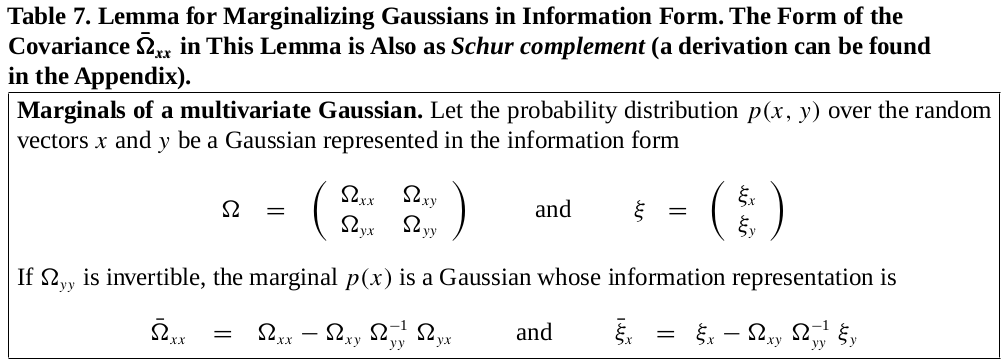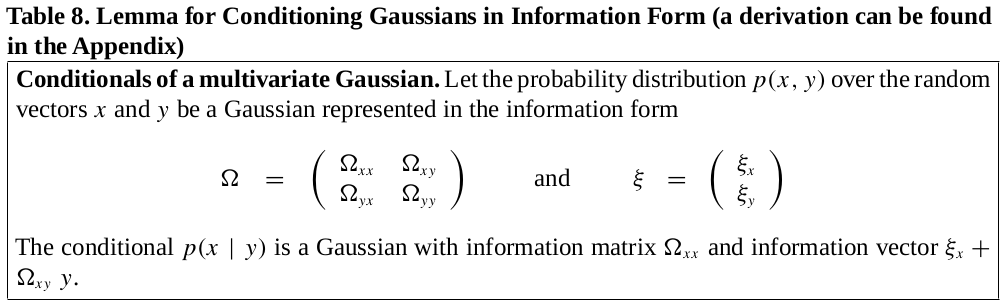0%

### 前言

GraphSLAM 的数学推导分为以下几步：

• 推导出一个关于 SLAM 全后验概率的递归公式
• 对每一个后验概率进行单独分析其对 SLAM 问题的影响
• 推导回复机器人位姿和地图特征点必要的公式

### SLAM 后验概率

• $z_{1:t}$ 是观测值
• $c_{1:t}$ 是观测值观测到特征点的序号
• $u_{1:t}$ 是控制输入量

### 负对数后验概率

• 机器人运动模型的概率服从 $\mathcal{N}(g(ut, x{t-1}), R_t)$，其中 $g$ 是运动模型函数，是确定性的；$R_t$ 则是运动误差的协方差矩阵
• 观测模型服从 $\mathcal{N}(h(x_t, c_t^i, i), W_t)$，其中 $h$ 是观测模型函数，是确定性的；$Q_t$ 则是观测误差的协方差矩阵

### 构建信息矩阵

• 先验 ：通过二次项 $x_0^T\Omega_0 x_0$，进行初始化：
• 控制量：从上式中，我们可以发现控制量 $u_t$ 对整体信息矩阵和信息向量的更新如下：
• 观测：同样，观测量 $z_t^i$ 对整体信息矩阵和信息向量的更新如下：

### 信息矩阵简化### 求解机器人路径和地图